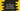# C program to find the factorial of a number using recursion### C program to find factorial of a number using recursion :

In this tutorial, we will learn how to find the factorial of a number in c programming language using recursion. Recursion means the function will call itself. For example, the factorial of 5 is 5 * 4 * 3 * 2 * 1. We will send this number to a function. It will call itself with 5 - 1 =4 and multiply it with 5. The inner function again call itself etc. It will be more meaningful if I explain this to you with an example. Let’s take a look into the program first :

### C program :

``````#include <stdio.h>

//4
int factorial(int n)
{
//5
if (n == 1)
return 1;
else
return (n * factorial(n - 1)); //6
}

int main()
{
//1
int no;

//2
printf("Enter a number : ");
scanf("%d", &no);

//3
int fact = factorial(no);

//7
printf("Factorial of %d is %d \n", no, fact);
}``````

### Explanation :

The commented numbers in the above program defines the steps below :

1. Create one variable no.
2. Take the number as input from the user and save it in no.
3. Now calculate the factorial. It calls int factorial(int n) method to find the factorial value. This function takes one int as input and returns one integer.
4. For example, if we want to find the factorial of 4,
• first function factorial will be called with 4 as input.
• First function will return 4 * fact(3)
• fact(3) returns 3 * fact(2)
• fact(2) returns 2 * fact(1)
• fact(1) returns 1 becase we have the if statement at first to return 1 if n == 1.

That means, first function will return 4 * fact(3) = 4 * 3 * fact(2) = 4 * 3 * 2 * fact(1) = 4 * 3 * 2 * 1 = 24 . Which is the factorial of 4

1. Finally, print out the result.

### Sample output :

``````Enter a number : 10
Factorial of 10 is 3628800

Enter a number : 1
Factorial of 1 is 1

Enter a number : 2
Factorial of 2 is 2

Enter a number : 5
Factorial of 5 is 120
``````